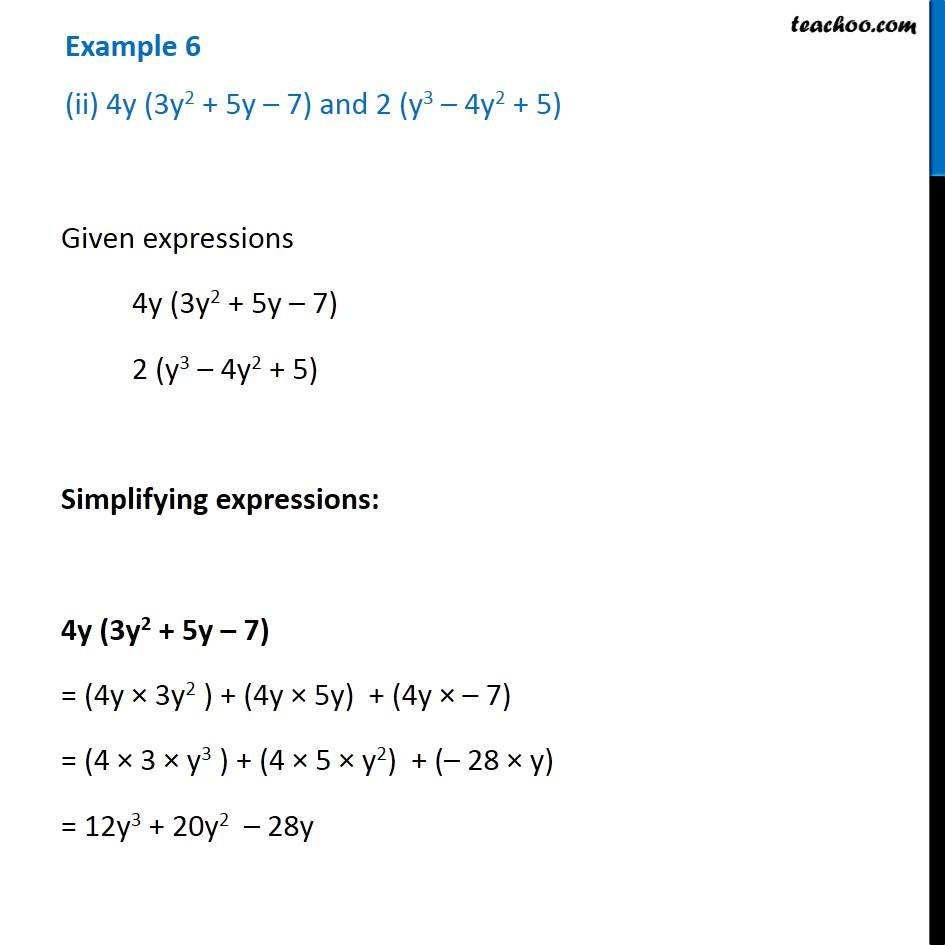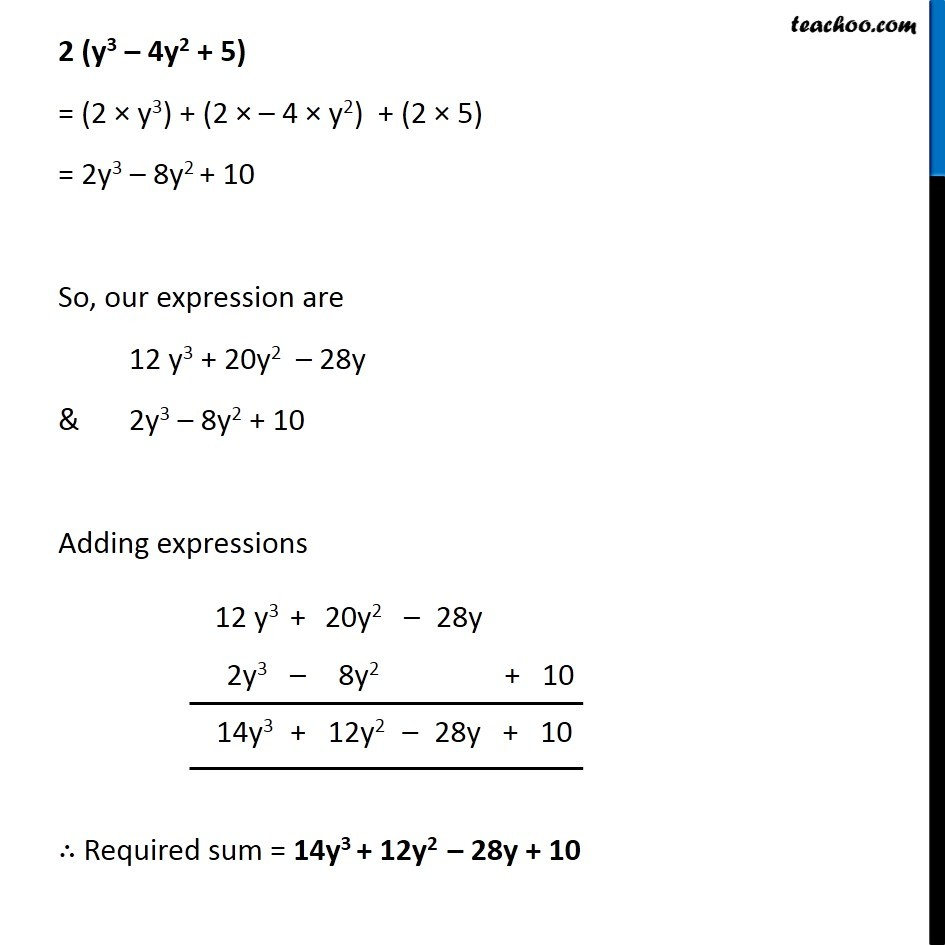Examples

Chapter 8 Class 8 Algebraic Expressions and Identities
Serial order wiseLearn in your speed, with individual attention - Teachoo Maths 1-on-1 Class

### Transcript

Example 6 (ii) 4y (3y2 + 5y – 7) and 2 (y3 – 4y2 + 5) Given expressions 4y (3y2 + 5y – 7) 2 (y3 – 4y2 + 5) Simplifying expressions: 4y (3y2 + 5y – 7) = (4y × 3y2 ) + (4y × 5y) + (4y × – 7) = (4 × 3 × y3 ) + (4 × 5 × y2) + (– 28 × y) = 12y3 + 20y2 – 28y 2 (y3 – 4y2 + 5) = (2 × y3) + (2 × – 4 × y2) + (2 × 5) = 2y3 – 8y2 + 10 So, our expression are 12 y3 + 20y2 – 28y & 2y3 – 8y2 + 10 Adding expressions ∴ Required sum = 14y3 + 12y2 – 28y + 10